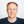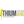0%
0%

using this we can keep track on our daily power usages and draw google graph for further analysis

Similar projects worth following
689 views
Since last few weeks i was thinking about making a project which can help me in keeping a track on my daily energy usages. In this project i am using a 30A current monitoring board. this board has 6 current sensor which can read upto 30A current. this board comes with an I2C interface and has 4 address lines so you can hookup up to 16 boards on the same I2C master device, which will give you total 96 current monitoring sensors.

I will also explain how you can write data into an xl sheet and in a google sheet using arduino.

Hardware you need

1. Arduino current monitoring

2. Arduino Nano hat

3. I2C cable

to read the current and power calculation i am using an arduino nano with an I2C adapter. This I2C adapter makes easy to connect current monitoring board.

I will also explain how you can write data into an xl sheet and in a google sheet using arduino.

Hardware you need

in this code i will write a command to read current through I2C.

this code is pretty straight forward, all you need to do is send a command to read current and the Current monitoring board will do everything for you.

In my area the AC voltage is around 120-124V. we will use this voltage reading to calculate the power uses.

in this code i am calculating current,power, watt hour, total watt hour and total kilo watt hour.

```//Include Wire I2C Library
#include  <wire.h>
double voltage = 121.7;   //////// voltage reading
double tkWh = 0;
double tWh = 0;
void setup()
{

Serial.begin(9600);  // Create Serial Object (9600 Baud)
Wire.begin();
}
void loop()
{

Wire.write(1);  // Command 1
Wire.write(1);  // Start Channel no
Wire.write(1);  // End Channel no
Wire.write(0);
Wire.write(0);
Wire.write((146 + 106 + 1 + 1 + 1 + 0 + 0) & 255); // CheckSum
Wire.endTransmission(); // Complete Transmission

MSB1= MSB1*65536;
MSB=MSB*256;
MSB1=MSB1+MSB+LSB;
double current = ((double)MSB1)/(double)1000;

Serial.print(current,3); //// current on ch 1
Serial.print(",");
double Power = voltage * current;  /// power on ch1
Serial.print(Power,3);
Serial.print(",");
double Wh = 0.000833 * Power;  /// Watt hour on ch1
Serial.print(Wh,4);
Serial.print(",");

tWh = tWh + Wh;   /// total watt hour used
Serial.print(tWh,4);
Serial.print(",");

tkWh = tWh/1000;  /// total kilo watt hour used
Serial.print(tkWh,4);
</p><p>
Serial.print("\n");

Wire.endTransmission(); // Complete Transmission

delay(3000);
}```

after reading the current now we will calculate the power.

power calculations are done in this section of the code.

All you need to do is burn the code in arduino and check your readings on serial port.

```Serial.print(current,3); //// current on ch 1

Serial.print(",");
double Power = voltage * current;  /// power on ch1
Serial.print(Power,3);
Serial.print(",");
double Wh = 0.000833 * Power;  /// Watt hour on ch1
Serial.print(Wh,4);
Serial.print(",");

tWh = tWh + Wh;   /// total watt hour used
Serial.print(tWh,4);
Serial.print(",");

tkWh = tWh/1000;  /// total killo watt hour used
Serial.print(tkWh,4);```

Just for the testing purpose i changed to a different different load just to see if it works with other load, so far it worked great.

you can also find the code on git.

Power monit using arduino

To write data into a text file i am using CoolTerm, its a really simple tool for capturing data.

to setup cool term

2. go to connection >> option

3. select serial port >> port

4. go to connection >> capture to text file

once you setup this it will start writing all the data coming from the arduino into a text file. We will use this data to plot power usages graph.

CoolTerm will save all data in a local folder and you can import this file into a XL sheet.

I separated different different readings with a "," so that i can create tables into the XL sheet. i will suggest if you have multiple data, do the same.

Now we will import the arduino data into a google sheet.

3. create a new google sheet

4. go to file import and select your arduino o/p text file

### current_xlsheet.ino

Power monitoring using arduino and Writing data in a text file using arduino and CoolTerm

ino - 1.78 kB - 06/23/2016 at 19:24

• 1 ×
• 1 × https://www.controleverything.com/content/I2C-Master?sku=ANI2C Arduino I2C shield
• 1 × Atmega32 Microprocessors, Microcontrollers, DSPs / ARM, RISC-Based MicrocontrollersAtmel
• 1 × Atmega128 Microprocessors, Microcontrollers, DSPs / ARM, RISC-Based Microcontrollers

Share

## Similar Projects

Project Owner ContributorJasper Sikken

Project Owner Contributor

### Another Precision Wattmeter Module INA219Lithium ION

Project Owner ContributorJasper Sikken